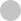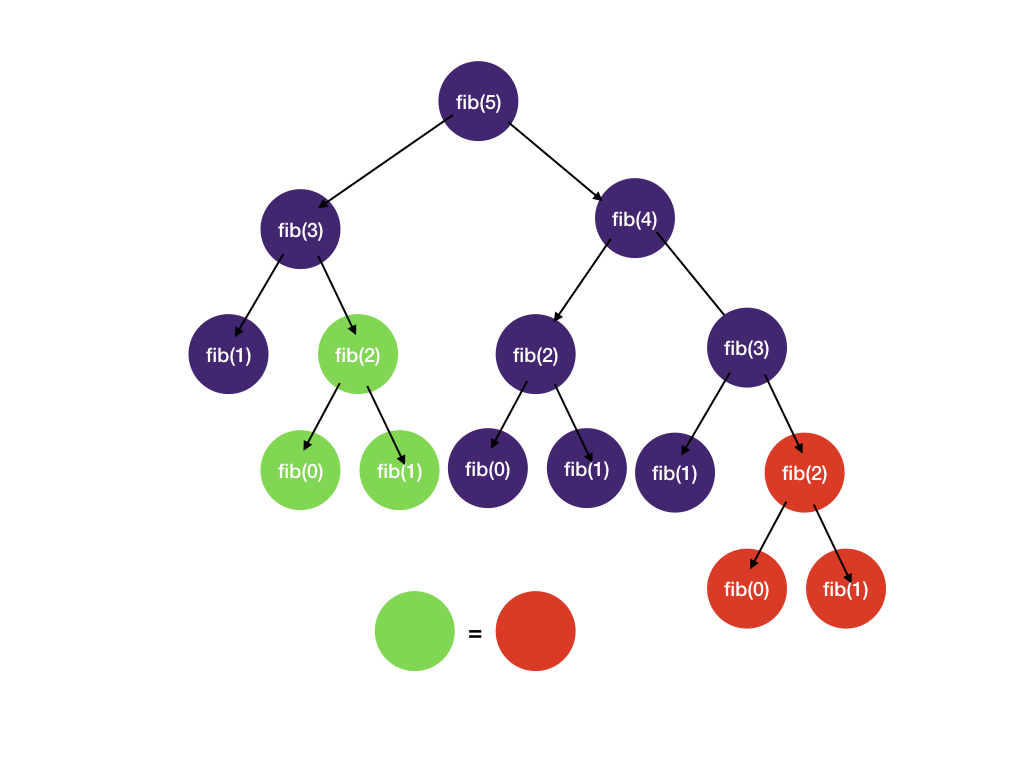#### Progress

0%#### Basic Data Structures#### Overview

•Vanilla Binary Search
•Finding Boundary

#### Sorted Array

•First Element Not Smaller Than Target 🔒
•First Occurrence
•Square Root 🔒

#### Implicitly Sorted Array#### Introduction

•Recursion Review
•DFS Fundamentals

#### Combinatorial Search

•DFS with States
•Backtracking Fundamentals
•Permutations
•Letter Combinations of Phone Number 🔒

#### Memoization

•Memoization Intro 🔒
•Word Break 🔒
•Decode Ways 🔒#### Introduction

•BFS Fundamentals

#### BFS on Tree#### Introduction

•Graph Fundamentals
•BFS on Graphs 🔒
•DFS on Graph 🔒
•BFS or DFS 🔒
•Matrix as Graph 🔒

#### BFS

•Shortest Path 🔒
•Word Ladder 🔒

#### Matrix as Graph

•Flood Fill 🔒
•Number of Islands 🔒
•Knight Minimum Moves 🔒

#### Directed Graph

•Course Schedule 🔒#### Same Direction

•Remove Duplicates 🔒
•Middle of Linked List 🔒
•Move Zeros 🔒

#### Opposite Direction

•Two Sum Sorted 🔒
•Valid Palindrome 🔒
•Trapping Rain Water 🔒

#### Prefix Sum

•Subarray Sum 🔒
•Subarray Sum Divisible by K 🔒#### Introduction

•Heap Fundamentals

#### Top K

•K Closest points 🔒
•Merge K Sorted Lists 🔒#### Binary Search#### Sequence

•House Robber 🔒
•Coin Change 🔒

#### Grid

•Number of Robot Paths 🔒
•Minimal Path Sum 🔒
•Maximal Square 🔒

#### Two Sequences

•Edit Distance 🔒
•Longest Common Subsequence 🔒

#### Game Theory

•Divisor Game 🔒#### Monotonic Stack

•Sliding Window Maximum

#### Data Structure Design

•LRU Cache 🔒# Dynamic Programming

Prerequisite: DFS, backtracking, memoization

## Dynamic what?

"Dynamic programming”, an awfully scary name. What does it even mean?? What’s so “dynamic” about programming?

The name was invented by Richard Bellman in the 1950s when computers are still decades away. So by “programming” he really did NOT mean programming as in coding at a computer. Bellman was a mathematician, and what he really meant by programming was “planning” and “decision making”.

Trivia time: according to Wikipedia, Bellman was working at RAND corporation, and it was hard to get mathematical research funding at the time. To disguise the fact that he was conducting mathematical research, he phrased his research in a less mathematical term “dynamic programming”. “The word dynamic was chosen by Bellman to capture the time-varying aspect of the problems, and because it sounded impressive. The word programming referred to the use of the method to find an optimal program, in the sense of a military schedule for training or logistics.”

So what he really meant was “multistage planning”, a simple concept of solving bigger problems using smaller problems while saving results to avoid repeated calculations. That sounds awfully familiar, isn’t that memoization? Yes it is. Keep on reading.

## What is Dynamic Programming?

A problem is a dynamic programming problem if it satisfy two conditions:

1) The problem can be divided into subproblems, and its optimal solution can be constructed from optimal solutions of the subproblems. In academic terms, this is called optimal substructure

2) The subproblems from 1) overlap.

## 1. Optimal substructure

Consider the problem of the shortest driving path from San Francisco (SF) to San Diego (SD). Since the highway goes through Los Angeles (LA), the problem can be divided into two subproblems - driving from SF to LA and driving from LA to SD.

In addition, shortest_path(SF, SD) = shortest_path(SF, LA) + shortest_path(LA, SD). Optimal solution to the problem = combination of optimal solutions of the subproblems.Now let’s look at an example where the problem does NOT have optimal substructure. Consider buying an airline ticket from New York (NYC) to San Francisco (SF). Let’s assume there is no direct flight and we have to transit through Chicago (CHI). Even though our trip is divided into two parts NYC to CHI and CHI to SF, most often the cheapest ticket from NYC to SF != cheapest ticket from NYC to CHI + cheapest ticket from CHI to SF because airlines do not normally price multi-leg trips the sum of individual flights to maximize profit.

## 2. Overlapping subproblems

As we have seen in the memoization section, Fibonacci number calculation has a good amount of repeated computation (overlapping subproblems) whose results can be cached and reused.If the above two conditions are satisfied, then the problem can be solved with dynamic programming.

## DP == DFS + memoization

You might have seen posts on coding forum titled “simple DFS solution” and “0.5 sec DP solution” for the same problem. The reason is the two methods are equivalent. They are two different approaches to DP: one is top-down, the other one is bottom-up.

## Two ways of Dynamic Programming

Top-down: this is basically DFS + memoization as we have seen memoization. We split large problems and recursively solving smaller subproblems.

Bottom-up: we try to solve subproblems first and then use their solutions to find solutions to bigger subproblems. This is normally done in a tabular form.

Let’s look at a concrete example.

## Fibonacci

Let's revisit the Fibonacci number problem from the memoization section.

### Top-down

Recall we have a system for backtracking and memoization

1. Draw the tree: see the tree above

2. Identify states

• What state do we need to know whether we have reached a solution? We need to know the value of `n` we are computing
• What state do we need to decide which child nodes should be visited next and which ones? There is no extra state we need. We always visit `n-1` and `n-2`.
1. DFS + memoization
``````def fib(n, memo):
if n in memo: # check in memo, if found, retrieve and return right away
return memo[n]

if n == 0 or n == 1:
return n

res = fib(n - 1, memo) + fib(n - 2, memo)

memo[n] = res # save in memo before returning
return res``````

### Bottom up

For the bottom-up dynamic programming, we want to start with subproblems first and work our way up to the main problem. This is normally done by filling up a table.

For the Fibonacci problem, we want to fill a one-dimensional table `dp` where each entry at index `i` represents value of the Fibonacci number at index i. The last element of the array is the result we want to return.

The order of filling matters because we cannot calculate `dp[i]` before we filled `dp[i - 1]` and `dp[i - 2]`.

``````def fib(n):
dp = [0, 1]
for i in range(2, n + 1):
dp.append(dp[i - 1] + dp[i - 2])

return dp[-1]``````

## Subproblems

The formula `dp[i] = dp[i - 1] + dp[i - 2]` is called the recurrence relation. It is the key to solving any dynamic programing problem.

For the Fibonacci number problem, the relation is already given `dp[i] = dp[i - 1] + dp[i - 2]`. We will discuss the patterns of recurrence relation in the next section.

## Should I do top-down or bottom-up?

Top-down pros:

• The order of computing subproblems doesn't matter. For bottom-up, we have to fill the table in an order such that all the subproblems are solved first. For example, to fill `dp`, we have to have filled `dp` and `dp` first. For top-down, we can let recursion and memoization take care of the subproblems and therefore not worry about the order.
• Easier to reason for partition type of problems (how many ways are there to.., splitting a string into...), just do DFS and add memoization.

Bottom-up pros:

• Easier to reason the time complexity (since it's just the time to fill the table)
• No recursion, and thus no system stack overflow although not a huge concern for normal coding interviews.

From our experiences, deciding on top-down or bottom-up depends on the problem. Some types of problems are easier to reason and solve with top-down than bottom-up and vice versa. We will see a breakdown in the next section.

## When to use dynamic programming

Mathematically, dynamic programming is an optimization method on one or more sequences (e.g. arrays, matrices). So questions asking about optimal way to do something on one or more sequences is often a good candidate for dynamic programming. Signs of dynamic programming:

• The problem asks for the maximum/longest, minimal/shortest value/cost/profit you can get from doing operations on a sequence.
• You've tried greedy but it sometimes it gives the wrong solution. This often means you have to consider subproblems for an optimal solution.
• The problem asks for how many ways there are to do something. This can often be solved by DFS + memoization, i.e. top-down dynamic programming.
• Partition a string/array into sub-sequences so that certain condition is met. This is often well-suited for top-down dynamic programming.
• The problems is about optimal way to play a game.

## Types of dynamic programming problems

Let's look at the types of dynamic programming problems in the next section. Keep on reading.